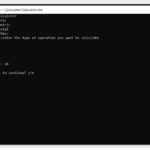## Advance Calculator In C++ With Source Code

The Advance Calculator is based on the concept of different mathematical calculations. This whole system is in the C++ language. Here, the user can perform various calculations like arithmetic, trigonometric, exponential, and logarithmic. Also, it is a ve...

Type : Project

File Size : 460.1 KB# Similar Projects and Reports

#### Advance Calculator In C++ With Source Code

The Advance Calculator is based on the concept of different mathematical calculations. This whole system is in the C++ language. Here, the user can perform various calculations like arithmetic, trigonometric, exponential, and logarithmic. Also, it is a ve...

#### ADVANCE CALCULATOR IN PHP WITH SOURCE CODE

The Advance Calculator In PHP is a simple project for solving the mathematical calculations of different numbers. You can see the basic features of the scientific calculator in this project.

#### Advance Console Calculator In C# With Source Code

Advance Console Calculator in C# is a simple project on the concept of normal mathematical operations on the calculator. The project contains the numbers, operators, and signs like in a normal calculator. Here, the user has to select the operation at firs...

#### CALCULATOR IN C++ WITH SOURCE CODE

This project is based on a concept to generate the total sum, subtractions, divisions and multiplication. User has to input numbers with mathematical signs (“+,-,*,/”), the system will display the output. [ user has to input : 5*5, then the system will d...

#### Calculator App In C# With Source Code

This Calculator App in C# is based on the concept of normal mathematical operations on the calculator. The project contains the numbers, operators, and signs like in a normal calculator. Here, the user cannot enter the number themselves, they can just cli...

#### Simple Calculator In C# With Source Code

Simple Calculator in C# is based on the concept of normal mathematical operations on the calculator. The project contains the numbers, operators, and signs like in a normal calculator. So, the user cannot enter the number themselves, they can just click o...